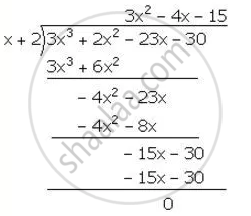Share

Using the Remainder Theorem, Factorise Each of the Following Completely. 3x3 + 2x2 – 23x – 30 - ICSE Class 10 - Mathematics

Question

Using the Remainder Theorem, factorise each of the following completely.

3x3 + 2x2 – 23x – 30

Solution

f(x)=3x^3+2x^2-23x-30

for X=-2,

f(x)=f(-2)=3(-2)^3+2(-2)^2-23(-2)-30

=-24+8+46-30=-54+54=0

Hence,(x+2) is a facctor of f(x)∴ 3x^3+2x^2-23x-30=(x+2)(3x^2-4x-15)

=(x+2)(3x^2+5x-9x-15)

=(x+2)[x(3x+5)-3(3x+5)]

=(x-2)(3x+5)(x-3)

Is there an error in this question or solution?

Video TutorialsVIEW ALL 

Solution Using the Remainder Theorem, Factorise Each of the Following Completely. 3x3 + 2x2 – 23x – 30 Concept: Factor Theorem.
S